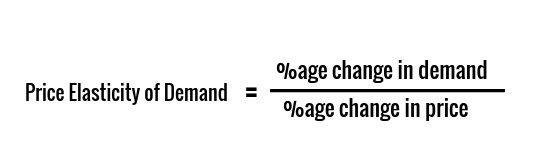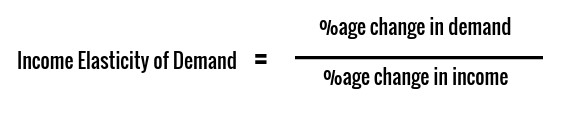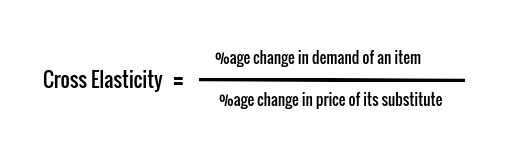# Elasticity of Demand

The elasticity of demand (PED or Ed), or elasticity, is the degree to which the demand for goods/services changes with change in
(a) Price of the goods/Service
(b) The income of the buyer
(c) Change in price of substitutes of goods/services.

#### Examples:

• If the price of apples rises, people will buy fewer apples, and hence its demand will fall.
• If the income of a person rises, he will buy more, and hence demand increases.
• If the price of coffee decreases, people will buy more coffee and less tea. So, with the rise in the price of coffee, the demand for tea decreases.

### The elasticity of demand is of three types:

#### Price Elasticity:

• Price Elasticity is defined as the percentage change in the demand for an item with a unit percentage change in the price of that item. Price elasticity is represented by EP.#### Income Elasticity:

• Income Elasticity is defined as the percentage change in demand for an item with a unit percentage change in income.#### Cross Elasticity:

• Cross Elasticity is defined as the percentage change in the demand for an item(X) with the unit percentage change in the price of its substitute/complement(Y).